Anzeige

26. Dec 2014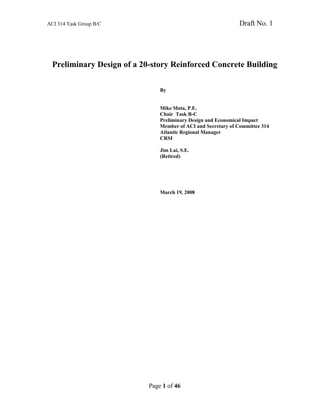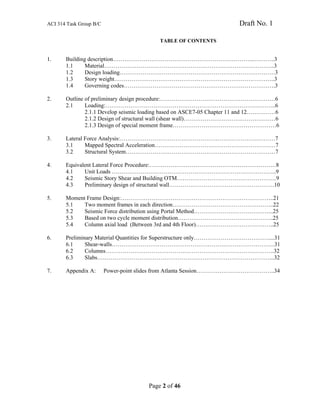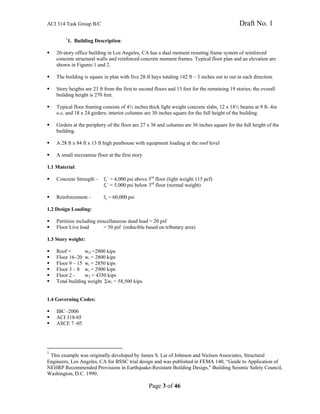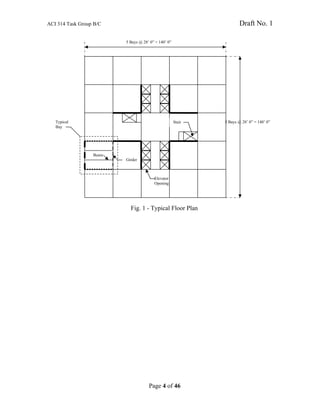Anzeige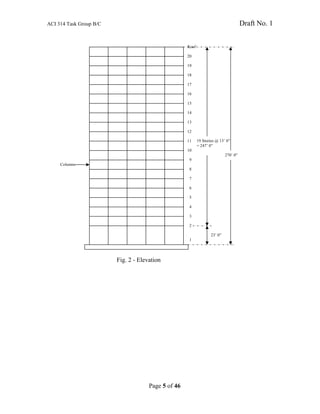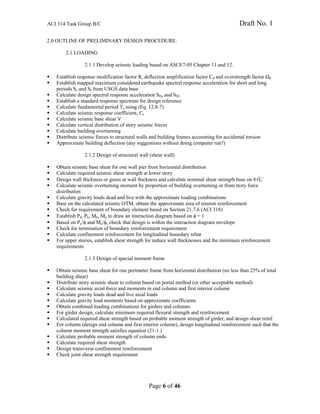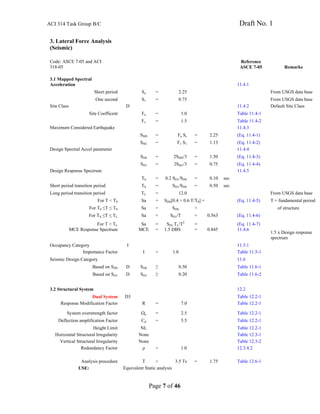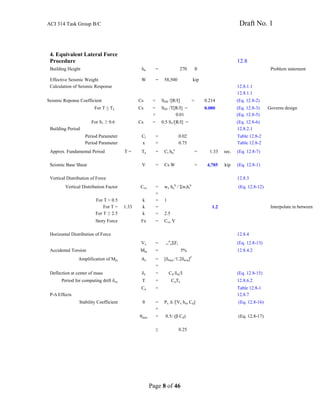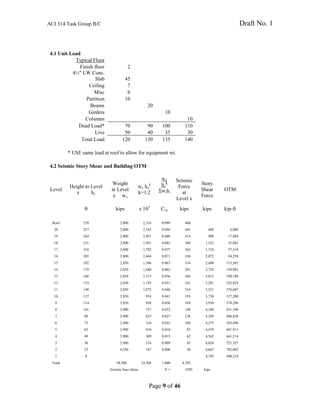Anzeige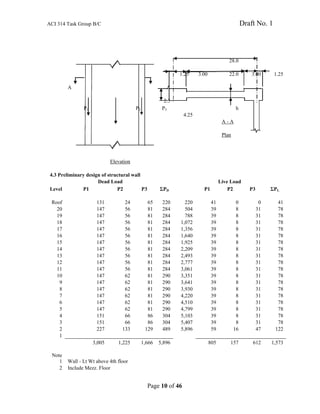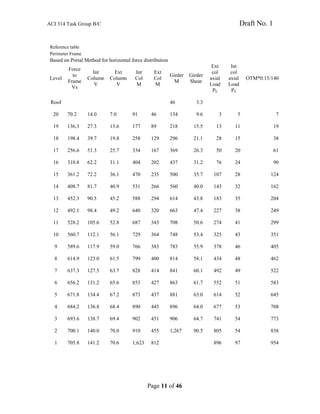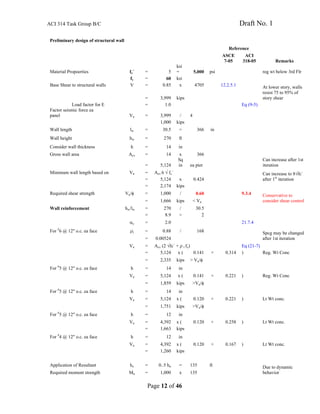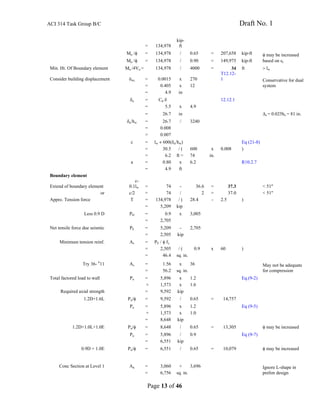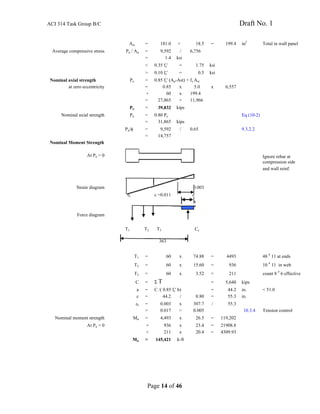Anzeige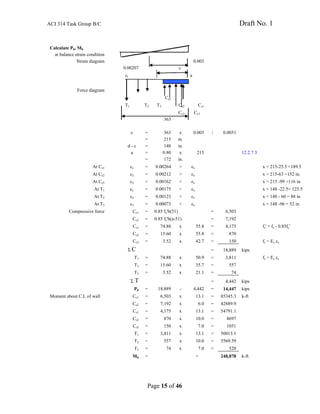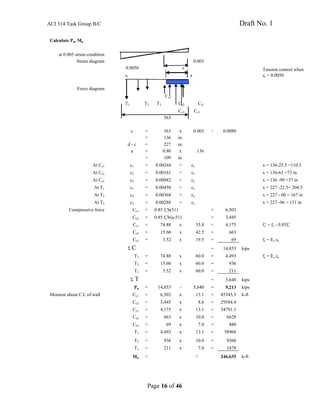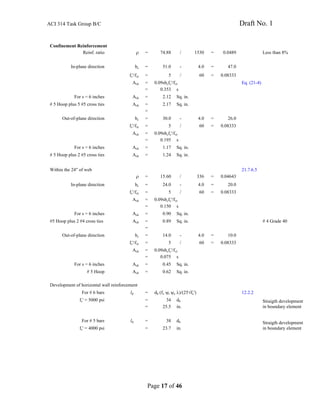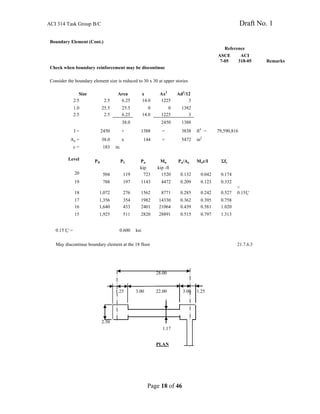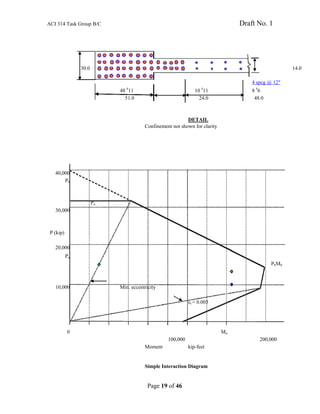Anzeige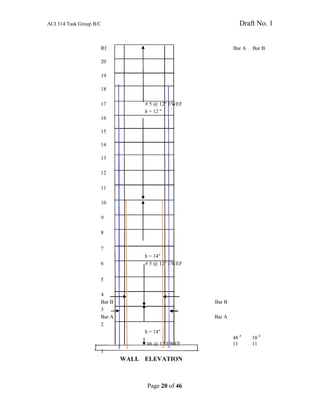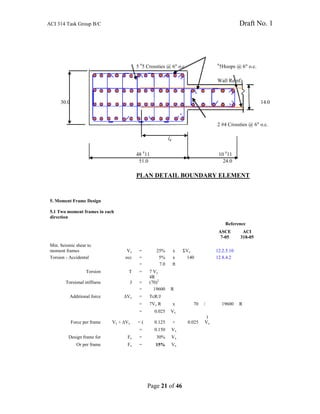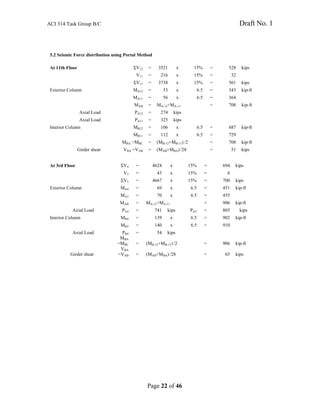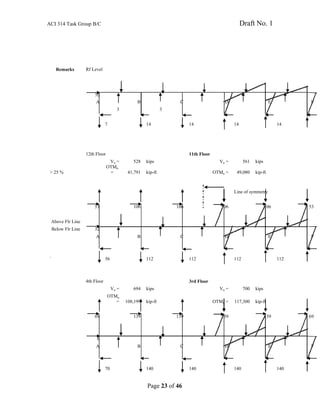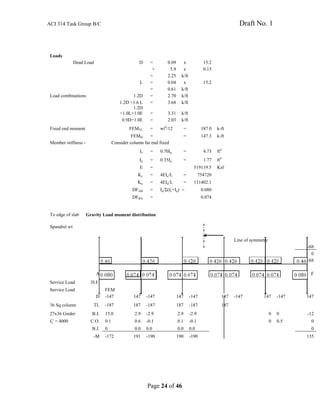Anzeige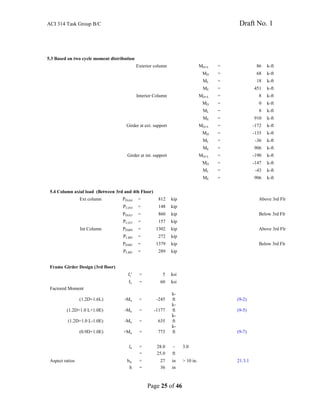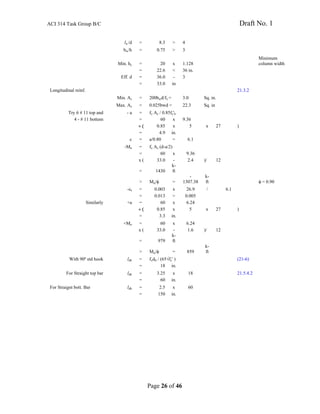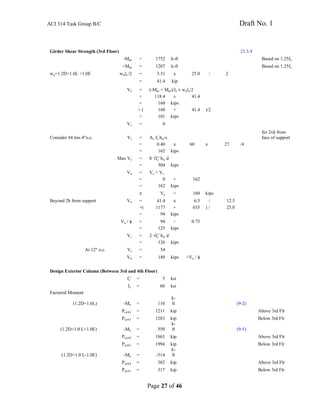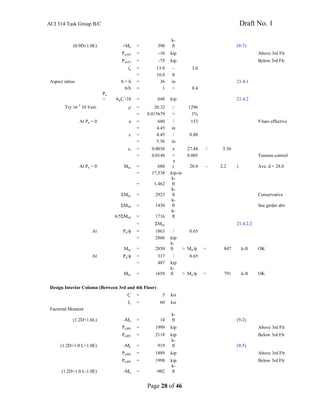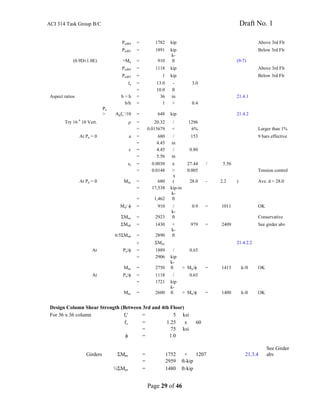Anzeige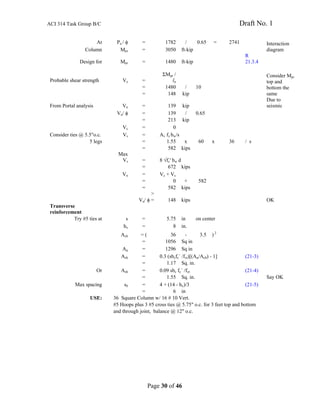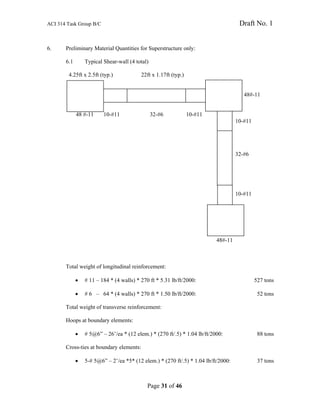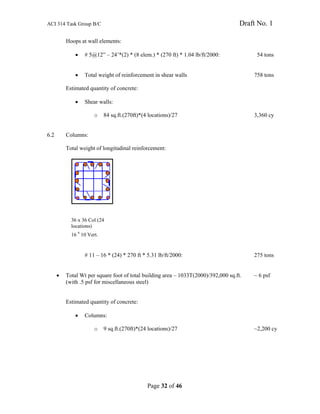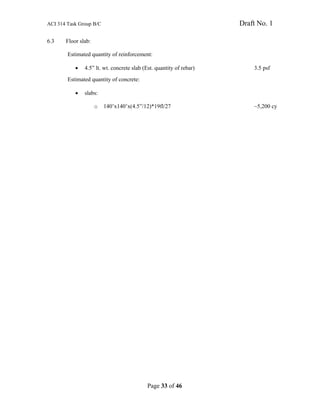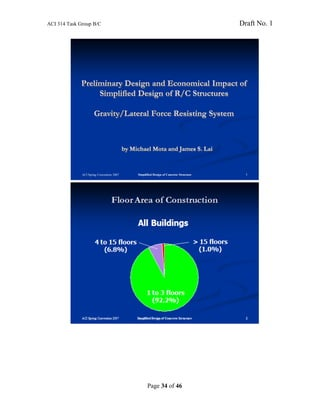Anzeige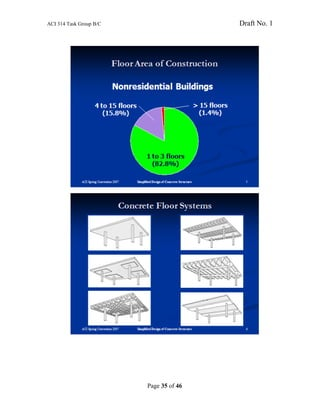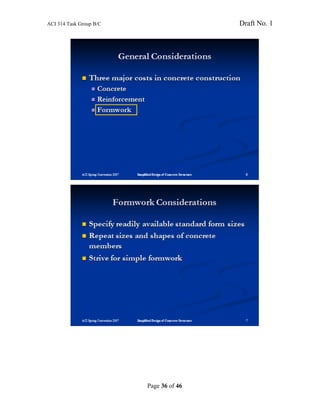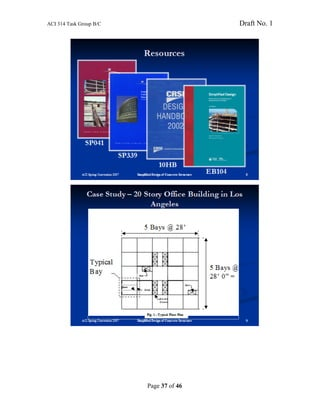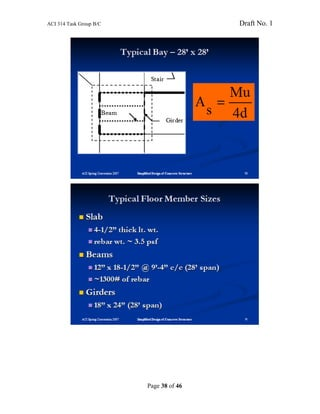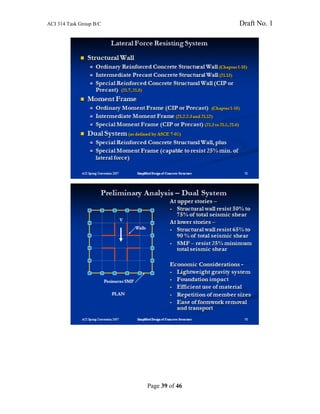Anzeige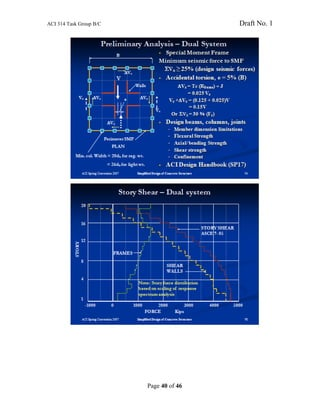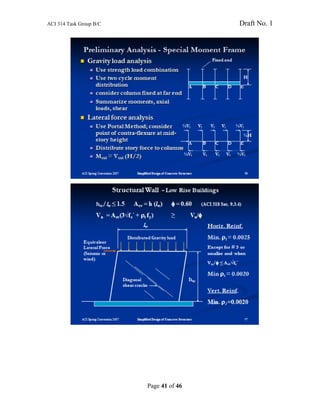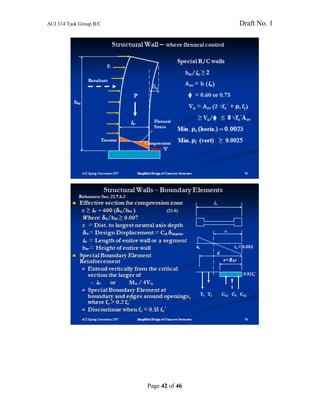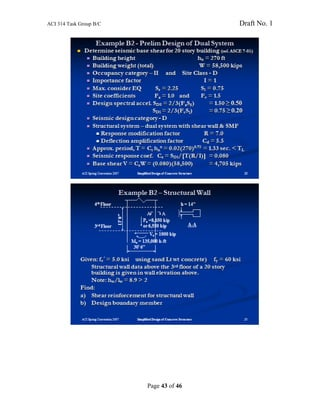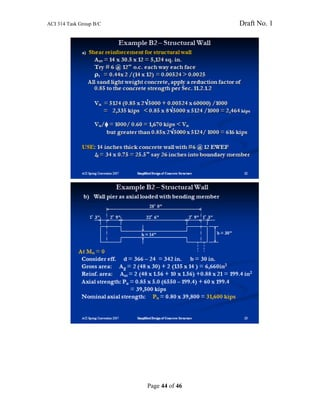Anzeige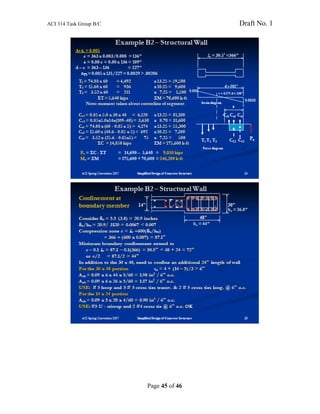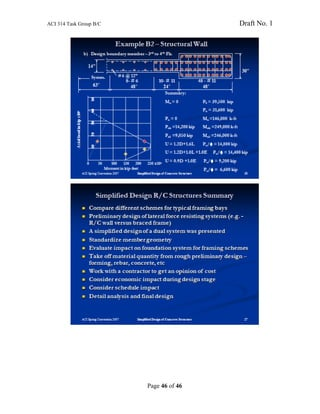Nächste SlideShare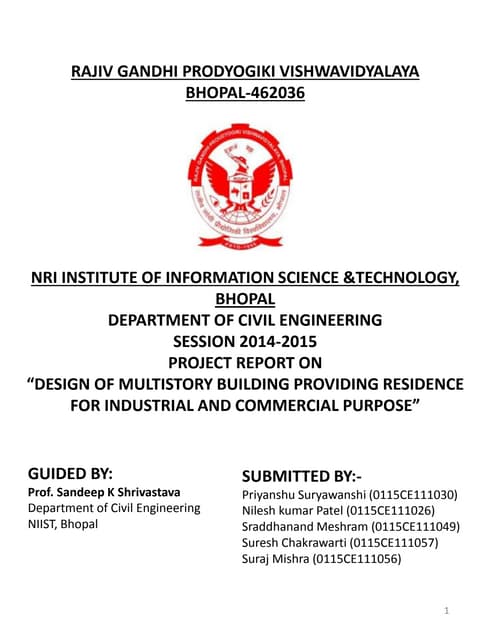Design and analasys of a g+2 residential building
1 von 46
Anzeige

1. ACI 314 Task Group B/C Draft No. 1 Page 1 of 46 Preliminary Design of a 20-story Reinforced Concrete Building By Mike Mota, P.E. Chair Task B-C Preliminary Design and Economical Impact Member of ACI and Secretary of Committee 314 Atlantic Regional Manager CRSI Jim Lai, S.E. (Retired) March 19, 2008
3. ACI 314 Task Group B/C Draft No. 1 Page 3 of 46 1 1. Building Description: 20-story office building in Los Angeles, CA has a dual moment resisting frame system of reinforced concrete structural walls and reinforced concrete moment frames. Typical floor plan and an elevation are shown in Figures 1 and 2. The building is square in plan with five 28-ft bays totaling 142 ft – 3 inches out to out in each direction. Story heights are 23 ft from the first to second floors and 13 feet for the remaining 19 stories; the overall building height is 270 feet. Typical floor framing consists of 4½ inches thick light weight concrete slabs, 12 x 18½ beams at 9 ft- 4in o.c. and 18 x 24 girders; interior columns are 30 inches square for the full height of the building. Girders at the periphery of the floor are 27 x 36 and columns are 36 inches square for the full height of the building. A 28 ft x 84 ft x 13 ft high penthouse with equipment loading at the roof level A small mezzanine floor at the first story 1.1 Material: Concrete Strength – fc´ = 4,000 psi above 3rd floor (light weight 115 pcf) fc´ = 5,000 psi below 3rd floor (normal weight) Reinforcement - fy = 60,000 psi 1.2 Design Loading: Partition including miscellaneous dead load = 20 psf Floor Live load = 50 psf (reducible based on tributary area) 1.3 Story weight: Roof = wrf =2800 kips Floor 16–20 wi = 2800 kips Floor 9 – 15 wi = 2850 kips Floor 3 – 8 wi = 2900 kips Floor 2 - w2 = 4350 kips Total building weight Σwi = 58,500 kips 1.4 Governing Codes: IBC -2006 ACI 318-05 ASCE 7 -05 1 This example was originally developed by James S. Lai of Johnson and Nielsen Associates, Structural Engineers, Los Angeles, CA for BSSC trial design and was published in FEMA 140, “Guide to Application of NEHRP Recommended Provisions in Earthquake-Resistant Building Design,” Building Seismic Safety Council, Washington, D.C. 1990.
4. ACI 314 Task Group B/C Draft No. 1 Page 4 of 46 Elevator Opening Beam Stair Girder Typical Bay 5 Bays @ 28’ 0” = 140’ 0” 5 Bays @ 28’ 0” = 140’ 0” Fig. 1 - Typical Floor Plan
5. ACI 314 Task Group B/C Draft No. 1 Page 5 of 46 Roof 20 19 18 17 16 15 14 13 12 11 10 9 8 7 6 5 4 3 2 1 19 Stories @ 13’ 0” = 247’ 0” 23’ 0” 270’ 0” Fig. 2 - Elevation Columns
7. ACI 314 Task Group B/C Draft No. 1 Page 7 of 46 3. Lateral Force Analysis (Seismic) Code: ASCE 7-05 and ACI 318-05 Reference ASCE 7-05 Remarks 3.1 Mapped Spectral Acceleration 11.4.1 Short period Sa = 2.25 From USGS data base One second S1 = 0.75 From USGS data base Site Class D 11.4.2 Default Site Class Site Coefficent Fa = 1.0 Table 11.4-1 Fv = 1.5 Table 11.4-2 Maximum Considered Earthquake 11.4.3 SMS = Fa Ss = 2.25 (Eq. 11.4-1) SM1 = Fv S1 = 1.13 (Eq. 11.4-2) Design Spectral Accel parameter 11.4.4 SDS = 2SMS/3 = 1.50 (Eq. 11.4-3) SD1 = 2SM1/3 = 0.75 (Eq. 11.4-4) Design Response Spectrum 11.4.5 T0 = 0.2 SD1/SDS = 0.10 sec Short period transition period TS = SD1/SDS = 0.50 sec Long period transition period TL = 12.0 From USGS data base For T < T0 Sa = SDS[0.4 + 0.6 T/T0] = (Eq. 11.4-5) T = fundamental period For T0 ≤T ≤ TS Sa = SDS = of structure For TS ≤T ≤ TL Sa = SD1/T = 0.563 (Eq. 11.4-6) For T > TL Sa = SD1 TL/T2 = (Eq. 11.4-7) MCE Response Spectrum MCE = 1.5 DBS = 0.845 11.4.6 1.5 x Design response spectrum Occupancy Category I 11.5.1 Importance Factor I = 1.0 Table 11.5-1 Seismic Design Category 11.6 Based on SDS D SDS ≥ 0.50 Table 11.6-1 Based on SD1 D SD1 ≥ 0.20 Table 11.6-2 3.2 Structural System 12.2 Dual System D3 Table 12.2-1 Response Modification Factor R = 7.0 Table 12.2-1 System overstrength factor Ωo = 2.5 Table 12.2-1 Deflection amplification Factor Cd = 5.5 Table 12.2-1 Height Limit NL Table 12.2-1 Horizontal Structural Irregularity None Table 12.3-1 Vertical Structural Irregularity None Table 12.3-2 Redundancy Factor ρ = 1.0 12.3.4.2 Analysis procedure T < 3.5 Ts = 1.75 Table 12.6-1 USE: Equivalent Static analysis
8. ACI 314 Task Group B/C Draft No. 1 Page 8 of 46 4. Equivalent Lateral Force Procedure 12.8 Building Height hn = 270 ft Problem statement Effective Seismic Weight W = 58,500 kip Calculation of Seismic Response 12.8.1.1 12.8.1.1 Seismic Reponse Coefficient Cs = SDS /[R/I] = 0.214 (Eq. 12.8-2) For T ≤ TL Cs = SD1 /T[R/I] = 0.080 (Eq. 12.8-3) Governs design > 0.01 (Eq. 12.8-5) For S1 ≥ 0.6 Cs = 0.5 S1/[R/I] = (Eq. 12.8-6) Building Period 12.8.2.1 Period Parameter Ct = 0.02 Table 12.8-2 Period Parameter x = 0.75 Table 12.8-2 Approx. Fundamental Period T = Ta = Ct hn x = 1.33 sec. (Eq. 12.8-7) Seismic Base Shear V = Cs W = 4,705 kip (Eq. 12.8-1) Vertical Distribution of Force 12.8.3 Vertical Distribution Factor Cvx = wx hx k / Σwihi k (Eq. 12.8-12) = For T < 0.5 k = 1 For T = 1.33 k = 1.2 Interpolate in between For T ≥ 2.5 k = 2.5 Story Force Fx = Cvx V Horizontal Distribution of Force 12.8.4 Vx = i= n xΣFi (Eq. 12.8-13) Accidental Torsion Mta = 5% 12.8.4.2 Amplification of Mta Ax = [δmax /1.2δavg]2 = Deflection at center of mass δx = Cd δse/I (Eq. 12.8-15) Period for computing drift δxe Τ = CuTa 12.8.6.2 Cu = Table 12.8-1 P-Δ Effects 12.8.7 Stability Coefficient θ = Px Δ /[Vx hsx Cd] (Eq. 12.8-16) = θmax = 0.5/ (β Cd) (Eq. 12.8-17) ≤ 0.25
9. ACI 314 Task Group B/C Draft No. 1 Page 9 of 46 4.1 Unit Load Typical Floor Finish floor 2 4½" LW Conc. Slab 45 Ceiling 7 Misc 6 Partition 10 Beams 20 Girders 10 Columns 10 Dead Load* 70 90 100 110 Live 50 40 35 30 Total Load 120 130 135 140 * USE same load at roof to allow for equipment wt. 4.2 Seismic Story Shear and Building OTM Level Height to Level x hx Weight at Level x wx wx hx k k=1.2 wx hx k Σwihi Seismic Force at Level x Story Shear Force OTM ft kips x 103 Cvx kips kips kip-ft Roof 270 2,800 2,316 0.099 468 20 257 2,800 2,183 0.094 441 468 6,080 19 244 2,800 2,051 0.088 414 908 17,889 18 231 2,800 1,921 0.082 388 1,323 35,083 17 218 2,800 1,792 0.077 362 1,710 57,319 16 205 2,800 1,664 0.071 336 2,072 84,258 15 192 2,850 1,566 0.067 316 2,408 115,565 14 179 2,850 1,440 0.062 291 2,724 150,983 13 166 2,850 1,315 0.056 266 3,015 190,180 12 153 2,850 1,193 0.051 241 3,281 232,829 11 140 2,850 1,072 0.046 216 3,521 278,607 10 127 2,850 954 0.041 193 3,738 327,200 9 114 2,850 838 0.036 169 3,930 378,296 8 101 2,900 737 0.032 149 4,100 431,590 7 88 2,900 625 0.027 126 4,248 486,820 6 75 2,900 516 0.022 104 4,375 543,690 5 62 2,900 410 0.018 83 4,479 601,913 4 49 2,900 309 0.013 62 4,562 661,214 3 36 2,900 214 0.009 43 4,624 721,327 2 23 4,350 187 0.008 38 4,667 782,002 1 0 4,705 890,218 Total 58,500 23,304 1.000 4,705 Seismic base shear V = 4705 kips
10. ACI 314 Task Group B/C Draft No. 1 Page 10 of 46 28.0 1.25 3.00 22.0 3.00 1.25 A A 2.5 P1 P2 P3 h 4.25 A - A Plan Elevation 4.3 Preliminary design of structural wall Dead Load Live Load Level P1 P2 P3 ΣPD P1 P2 P3 ΣPL Roof 131 24 65 220 220 41 0 0 41 20 147 56 81 284 504 39 8 31 78 19 147 56 81 284 788 39 8 31 78 18 147 56 81 284 1,072 39 8 31 78 17 147 56 81 284 1,356 39 8 31 78 16 147 56 81 284 1,640 39 8 31 78 15 147 56 81 284 1,925 39 8 31 78 14 147 56 81 284 2,209 39 8 31 78 13 147 56 81 284 2,493 39 8 31 78 12 147 56 81 284 2,777 39 8 31 78 11 147 56 81 284 3,061 39 8 31 78 10 147 62 81 290 3,351 39 8 31 78 9 147 62 81 290 3,641 39 8 31 78 8 147 62 81 290 3,930 39 8 31 78 7 147 62 81 290 4,220 39 8 31 78 6 147 62 81 290 4,510 39 8 31 78 5 147 62 81 290 4,799 39 8 31 78 4 151 66 86 304 5,103 39 8 31 78 3 151 66 86 304 5,407 39 8 31 78 2 227 133 129 489 5,896 59 16 47 122 1 3,005 1,225 1,666 5,896 805 157 612 1,573 Note 1 Wall - Lt Wt above 4th floor 2 Include Mezz. Floor
11. ACI 314 Task Group B/C Draft No. 1 Page 11 of 46 Reference table Perimeter Frame Based on Portal Method for horizontal force distribution Level Force to Frame Vs Int Column V Ext Column V Int Col M Ext Col M Girder M Girder Shear Ext col axial Load PE Int col axial Load PE OTM*0.15/140 Roof 46 3.3 20 70.2 14.0 7.0 91 46 134 9.6 3 5 7 19 136.3 27.3 13.6 177 89 218 15.5 13 11 19 18 198.4 39.7 19.8 258 129 296 21.1 28 15 38 17 256.6 51.3 25.7 334 167 369 26.3 50 20 61 16 310.8 62.2 31.1 404 202 437 31.2 76 24 90 15 361.2 72.2 36.1 470 235 500 35.7 107 28 124 14 408.7 81.7 40.9 531 266 560 40.0 143 32 162 13 452.3 90.5 45.2 588 294 614 43.8 183 35 204 12 492.1 98.4 49.2 640 320 663 47.4 227 38 249 11 528.2 105.6 52.8 687 343 708 50.6 274 41 299 10 560.7 112.1 56.1 729 364 748 53.4 325 43 351 9 589.6 117.9 59.0 766 383 783 55.9 378 46 405 8 614.9 123.0 61.5 799 400 814 58.1 434 48 462 7 637.3 127.5 63.7 828 414 841 60.1 492 49 522 6 656.2 131.2 65.6 853 427 863 61.7 552 51 583 5 671.8 134.4 67.2 873 437 881 63.0 614 52 645 4 684.2 136.8 68.4 890 445 896 64.0 677 53 708 3 693.6 138.7 69.4 902 451 906 64.7 741 54 773 2 700.1 140.0 70.0 910 455 1,267 90.5 805 54 838 1 705.8 141.2 70.6 1,623 812 896 97 954
12. ACI 314 Task Group B/C Draft No. 1 Page 12 of 46 Preliminary design of structural wall Reference ASCE 7-05 ACI 318-05 Remarks Material Propoerties fc´ = 5 ksi = 5,000 psi reg wt below 3rd Flr fy = 60 ksi Base Shear to structural walls V = 0.85 x 4705 12.2.5.1 At lower story, walls resist 75 to 95% of story shear= 3,999 kips Load factor for E = 1.0 Eq (9-5) Factor seismic force ea panel Vu = 3,999 / 4 1,000 kips Wall length lw = 30.5 = 366 in Wall height hw = 270 ft Consider wall thickness h = 14 in Gross wall area Acv = 14 x 366 Can increase after 1st iteration= 5,124 Sq in ea pier Minimum wall length based on Vn = Acv 6 √ fc´ Can increase to 8√fc´ after 1st iteration= 5,124 x 0.424 = 2,174 kips Required shear strength Vu/φ = 1,000 / 0.60 9.3.4 Conservative to consider shear control= 1,666 kips < Vn Wall reinforcement hw/lw = 270 / 30.5 = 8.9 > 2 αc = 2.0 21.7.4 For # 6 @ 12" o.c. ea face ρt = 0.88 / 168 Spcg may be changed after 1st iteration= 0.00524 Vn = Acv (2 √fc´ + ρ t fy) Eq (21-7) = 5,124 x ( 0.141 + 0.314 ) Reg. Wt Conc = 2,335 kips > Vu/φ For # 5 @ 12" o.c. ea face h = 14 in Vn = 5,124 x ( 0.141 + 0.221 ) Reg. Wt Conc = 1,859 kips >Vu/φ For # 5 @ 12" o.c. ea face h = 14 in Vn = 5,124 x ( 0.120 + 0.221 ) Lt Wt conc. = 1,751 kips >Vu/φ For # 5 @ 12" o.c. ea face h = 12 in Vn = 4,392 x ( 0.120 + 0.258 ) Lt Wt conc. = 1,663 kips For # 4 @ 12" o.c. ea face h = 12 in Vn = 4,392 x ( 0.120 + 0.167 ) Lt Wt conc. = 1,260 kips Application of Resultant hx = 0..5 hn = 135 ft Due to dynamic behaviorRequired moment strength Mu = 1,000 x 135
13. ACI 314 Task Group B/C Draft No. 1 Page 13 of 46 = 134,978 kip- ft Mu /φ = 134,978 / 0.65 = 207,658 kip-ft φ may be increased based on εtMu /φ = 134,978 / 0.90 = 149,975 kip-ft Min. Ht. Of Boundary element Mu /4Vu = 134,978 / 4000 = 34 ft > lw Consider building displacement δσε = 0.0015 x 270 T12.12- 1 Conservative for dual system= 0.405 x 12 = 4.9 in δu = Cd δ 12.12.1 = 5.5 x 4.9 = 26.7 in Δs = 0.025hx = 81 in. δu/hw = 26.7 / 3240 = 0.008 > 0.007 c = lw ÷ 600(δu/hw) Eq (21-8) = 30.5 / ( 600 x 0.008 ) = 6.2 ft = 74 in. a = 0.80 x 6.2 R10.2.7 = 4.9 ft Boundary element Extend of boundary element c- 0.1lw = 74 - 36.6 = 37.3 < 51" or c/2 = 74 / 2 = 37.0 < 51" Appro. Tension force T = 134,978 / ( 28.4 - 2.5 ) = 5,209 kip Less 0.9 D PD = 0.9 x 3,005 = 2,705 Net tensile force due seismic PE = 5,209 - 2,705 = 2,505 kip Minimum tension reinf. As = PE / φ fy = 2,505 / ( 0.9 x 60 ) = 46.4 sq. in. Try 36- # 11 As = 1.56 x 36 May not be adequate for compression= 56.2 sq. in. Total factored load to wall Pu = 5,896 x 1.2 Eq.(9-2) + 1,573 x 1.6 Required axial strength = 9,592 kip 1.2D+1.6L Pu/φ = 9,592 / 0.65 = 14,757 Pu = 5,896 x 1.2 Eq (9-5) + 1,573 x 1.0 = 8,648 kip 1.2D+1.0L+1.0E Pu/φ = 8,648 / 0.65 = 13,305 φ may be increased Pu = 5,896 / 0.9 Eq (9-7) = 6,551 kip 0.9D + 1.0E Pu/φ = 6,551 / 0.65 = 10,079 φ may be increased Conc Section at Level 1 Ag = 3,060 + 3,696 Ignore L-shape in prelim design= 6,756 sq. in.
14. ACI 314 Task Group B/C Draft No. 1 Page 14 of 46 Ast = 181.0 + 18.5 = 199.4 in2 Total in wall panel Average compressive stress Pu / Ag = 9,592 / 6,756 = 1.4 ksi < 0.35 fc' = 1.75 ksi > 0.10 fc' = 0.5 ksi Nominal axial strength Po = 0.85 fc' (Ag-Ast) + fy Ast at zero eccentricity = 0.85 x 5.0 x 6,557 + 60 x 199.4 = 27,865 + 11,966 Po = 39,832 kips Nominal axial strength Pn = 0.80 Po Eq (10-2) = 31,865 kips Pu/φ = 9,592 / 0.65 9.3.2.2 = 14,757 Nominal Moment Strength At Pn = 0 Ignore rebar at compression side and wall reinf. Strain diagram 0.003 εt ε =0.011 c a Force diagram T1 T2 T3 Cc 363 T1 = 60 x 74.88 = 4493 48 # 11 at ends T2 = 60 x 15.60 = 936 10 # 11 in web T3 = 60 x 3.52 = 211 count 8 # 6 effective C = Σ T = 5,640 kips a = C /( 0.85 fc' b) = 44.2 in. < 51.0 c = 44.2 / 0.80 = 55.3 in. εt = 0.003 x 307.7 / 55.3 = 0.017 > 0.005 10.3.4 Tension control Nominal moment strength Mn = 4,493 x 26.5 = 119,202 At Pn = 0 + 936 x 23.4 = 21908.8 + 211 x 20.4 = 4309.93 Mn = 145,421 k-ft
15. ACI 314 Task Group B/C Draft No. 1 Page 15 of 46 Calculate Pb, Mb at balance strain condition Strain diagram 0.003 0.00207 c εt a Force diagram Cs3 T1 T2 T3 Cs2 Cs1 Cc2 Cc1 363 c = 363 x 0.003 / 0.0051 = 215 in. d - c = 148 in. a = 0.80 x 215 12.2.7.3 = 172 in. At Cs1 ε1 = 0.00264 > εy x = 215-25.5 =189.5 At Cs2 ε2 = 0.00212 > εy x = 215-63 =152 in. At Cs3 ε3 = 0.00162 < εy x = 215 -99 =116 in At T1 ε1 = 0.00175 < εy x = 148 -22.5= 125.5 At T2 ε2 = 0.00123 < εy x = 148 - 60 = 88 in At T3 ε3 = 0.00073 < εy x = 148 -96 = 52 in Compressive force Cc1 = 0.85 fc'b(51) = 6,503 Cc2 = 0.85 fc'b(a-51) = 7,192 Cs1 = 74.88 x 55.8 = 4,175 fs' = fs - 0.85fc' Cs2 = 15.60 x 55.8 = 870 Cs3 = 3.52 x 42.7 = 150 fs = Es εs Σ C = 18,889 kips T1 = 74.88 x 50.9 = 3,811 fs = Es εs T2 = 15.60 x 35.7 = 557 T3 = 3.52 x 21.1 = 74 Σ T = 4,442 kips Pb = 18,889 - 4,442 = 14,447 kips Moment about C.L of wall Cc1 = 6,503 x 13.1 = 85345.3 k-ft Cc2 = 7,192 x 6.0 = 42889.9 Cs1 = 4,175 x 13.1 = 54791.1 Cs2 = 870 x 10.0 = 8697 Cs3 = 150 x 7.0 = 1051 T1 = 3,811 x 13.1 = 50013.1 T2 = 557 x 10.0 = 5569.59 T3 = 74 x 7.0 = 520 Mb = = 248,878 k-ft
16. ACI 314 Task Group B/C Draft No. 1 Page 16 of 46 Calculate Pn, Mn at 0.005 strain condition Strain diagram 0.003 0.0050 c Tension control when εt > 0.0050εt a Force diagram Cs3 T1 T2 T3 Cs2 Cs1 Cc2 Cc1 363 c = 363 x 0.003 / 0.0080 = 136 in. d - c = 227 in. a = 0.80 x 136 = 109 in. At Cs1 ε1 = 0.00244 > εy x = 136-25.5 =110.5 At Cs2 ε2 = 0.00161 < εy x = 136-63 =73 in. At Cs3 ε3 = 0.00082 < εy x = 136 -99 =37 in At T1 ε1 = 0.00450 > εy x = 227 -22.5= 204.5 At T2 ε2 = 0.00368 > εy x = 227 - 60 = 167 in At T3 ε3 = 0.00288 > εy x = 227 -96 = 131 in Compressive force Cc1 = 0.85 fc'b(51) = 6,503 Cc2 = 0.85 fc'b(a-51) = 3,445 Cs1 = 74.88 x 55.8 = 4,175 fs' = fs - 0.85fc' Cs2 = 15.60 x 42.5 = 663 Cs3 = 3.52 x 19.5 = 69 fs = Es εs Σ C = 14,853 kips T1 = 74.88 x 60.0 = 4,493 fs = Es εs T2 = 15.60 x 60.0 = 936 T3 = 3.52 x 60.0 = 211 Σ T = 5,640 kips Pn = 14,853 - 5,640 = 9,213 kips Moment about C.L of wall Cc1 = 6,503 x 13.1 = 85345.3 k-ft Cc2 = 3,445 x 8.6 = 29584.4 Cs1 = 4,175 x 13.1 = 54791.1 Cs2 = 663 x 10.0 = 6628 Cs3 = 69 x 7.0 = 480 T1 = 4,493 x 13.1 = 58968 T2 = 936 x 10.0 = 9360 T3 = 211 x 7.0 = 1478 Mn = = 246,635 k-ft
17. ACI 314 Task Group B/C Draft No. 1 Page 17 of 46 Confinement Reinforcement Reinf. ratio ρ = 74.88 / 1530 = 0.0489 Less than 8% In-plane direction bc = 51.0 - 4.0 = 47.0 fc'/fyt = 5 / 60 = 0.08333 Ash = 0.09sbcfc'/fyt Eq. (21-4) = 0.353 s For s = 6 inches Ash = 2.12 Sq. in. # 5 Hoop plus 5 #5 cross ties Ash = 2.17 Sq. in. = Out-of-plane direction bc = 30.0 - 4.0 = 26.0 fc'/fyt = 5 / 60 = 0.08333 Ash = 0.09sbcfc'/fyt = 0.195 s For s = 6 inches Ash = 1.17 Sq. in. # 5 Hoop plus 2 #5 cross ties Ash = 1.24 Sq. in. Within the 24" of web 21.7.6.5 ρ = 15.60 / 336 = 0.04643 In-plane direction bc = 24.0 - 4.0 = 20.0 fc'/fyt = 5 / 60 = 0.08333 Ash = 0.09sbcfc'/fyt = 0.150 s For s = 6 inches Ash = 0.90 Sq. in. #5 Hoop plus 2 #4 cross ties Ash = 0.89 Sq. in. # 4 Grade 40 = Out-of-plane direction bc = 14.0 - 4.0 = 10.0 fc'/fyt = 5 / 60 = 0.08333 Ash = 0.09sbcfc'/fyt = 0.075 s For s = 6 inches Ash = 0.45 Sq. in. # 5 Hoop Ash = 0.62 Sq. in. Development of horizontal wall reinforcement For # 6 bars ld = db (fy ψt ψe λ)/(25√fc') 12.2.2 fc' = 5000 psi = 34 db Straigth development in boundary element= 25.5 in. For # 5 bars ld = 38 db Straigth development in boundary elementfc' = 4000 psi = 23.7 in.
18. ACI 314 Task Group B/C Draft No. 1 Page 18 of 46 Boundary Element (Cont.) Reference ASCE 7-05 ACI 318-05 Remarks Check when boundary reinforcement may be discontinue Consider the boundary element size is reduced to 30 x 30 at upper stories Size Area x Ax2 Ad2 /12 2.5 2.5 6.25 14.0 1225 3 1.0 25.5 25.5 0 0 1382 2.5 2.5 6.25 14.0 1225 3 38.0 2450 1388 I = 2450 + 1388 = 3838 ft4 = 79,590,816 Ag = 38.0 x 144 = 5472 in2 c = 183 in. Level PD PL Pu Mu Pu/Ag Muc/I Σfc kip kip -ft 20 504 119 723 1520 0.132 0.042 0.174 19 788 197 1143 4472 0.209 0.123 0.332 18 1,072 276 1562 8771 0.285 0.242 0.527 < 0.15fc' 17 1,356 354 1982 14330 0.362 0.395 0.758 16 1,640 433 2401 21064 0.439 0.581 1.020 15 1,925 511 2820 28891 0.515 0.797 1.313 0.15 fc' = 0.600 ksi May discontinue boundary element at the 18 floor 21.7.6.3 28.00 1.25 3.00 22.00 3.00 1.25 2.50 1.17 PLAN
19. ACI 314 Task Group B/C Draft No. 1 Page 19 of 46 30.0 14.0 4 spcg @ 12" 48 # 11 10 # 11 8 # 6 51.0 24.0 48.0 DETAIL Confinement not shown for clarity 40,000 P0 Pn 30,000 P (kip) 20,000 Pn PbMb 10,000 Min. eccentricity εt = 0.005 0 Mn 100,000 200,000 Moment kip-feet Simple Interaction Diagram
20. ACI 314 Task Group B/C Draft No. 1 Page 20 of 46 Rf Bar A Bar B 20 19 18 17 # 5 @ 12" EWEF h = 12 " 16 15 14 13 12 11 10 9 8 7 h = 14" 6 # 5 @ 12" EWEF 5 4 Bar B Bar B 3 Bar A Bar A 2 h = 14" #6 @ 12 EWEF 48 # 11 10 # 11 1 WALL ELEVATION
21. ACI 314 Task Group B/C Draft No. 1 Page 21 of 46 5 # 5 Crossties @ 6" o.c. # 5Hoops @ 6" o.c. Wall Reinf. 30.0 14.0 2 #4 Crossties @ 6" o.c. ld 48 # 11 10 # 11 51.0 24.0 PLAN DETAIL BOUNDARY ELEMENT 5. Moment Frame Design 5.1 Two moment frames in each direction Reference ASCE 7-05 ACI 318-05 Min. Seismic shear to moment frames Vx = 25% x ΣVx 12.2.5.10 Torsion - Accidental ecc = 5% x 140 12.8.4.2 = 7.0 ft Torsion T = 7 Vx Torsional stiffness J = 4R (70)2 = 19600 R Additional force ΔVx = TcR/J = 7Vx R x 70 / 19600 R = 0.025 Vx Force per frame Vx + ΔVx = ( 0.125 + 0.025 ) Vx = 0.150 Vx Design frame for Fx = 30% Vx Or per frame Fx = 15% Vx
22. ACI 314 Task Group B/C Draft No. 1 Page 22 of 46 5.2 Seismic Force distribution using Portal Method At 11th Floor ΣV12 = 3521 x 15% = 528 kips V11 = 216 x 15% = 32 ΣV11 = 3738 x 15% = 561 kips Exterior Column MA12 = 53 x 6.5 = 343 kip-ft MA11 = 56 x 6.5 = 364 MAB = MA-12+MA-11 = 708 kip-ft Axial Load PA12 = 274 kips Axial Load PA11 = 325 kips Interior Column MB12 = 106 x 6.5 = 687 kip-ft MB11 = 112 x 6.5 = 729 MBA =MBC = (MB-12+MB-11) /2 = 708 kip-ft Girder shear VBA =VAB = (MAB+MBA) /28 = 51 kips At 3rd Floor ΣV4 = 4624 x 15% = 694 kips V3 = 43 x 15% = 6 ΣV3 = 4667 x 15% = 700 kips Exterior Column MA4 = 69 x 6.5 = 451 kip-ft MA3 = 70 x 6.5 = 455 MAB = MA-12+MA-11 = 906 kip-ft Axial Load PA4 = 741 kips PA3 = 805 kips Interior Column MB4 = 139 x 6.5 = 902 kip-ft MB3 = 140 x 6.5 = 910 Axial Load PB4 = 54 kips MBA =MBC = (MB-12+MB-11) /2 = 906 kip-ft Girder shear VBA =VAB = (MAB+MBA) /28 = 65 kips
23. ACI 314 Task Group B/C Draft No. 1 Page 23 of 46 Remarks Rf Level 70 A B C D E F 3 3 7 14 14 14 14 12th Floor 11th Floor Vu = 528 kips Vu = 561 kips > 25 % OTMu = 41,791 kip-ft OTMu = 49,080 kip-ft Line of symmetry 53 106 106 106 106 53 Above Flr Line Below Flr Line 32 A B C D E F ` 56 112 112 112 112 4th Floor 3rd Floor Vu = 694 kips Vu = 700 kips OTMu = 108,199 kip-ft OTMu = 117,300 kip-ft 69 139 139 139 139 69 6 A B C D E F 70 140 140 140 140
24. ACI 314 Task Group B/C Draft No. 1 Page 24 of 46 Loads Dead Load D = 0.09 x 15.2 + 5.9 x 0.15 = 2.25 k/ft L = 0.04 x 15.2 = 0.61 k/ft Load combinations 1.2D = 2.70 k/ft 1.2D +1.6 L = 3.68 k/ft 1.2D +1.0L+1.0E = 3.31 k/ft 0.9D+1.0E = 2.03 k/ft Fixed end moment FEMTL = wl2 /12 = 187.0 k-ft FEMD = = 147.3 k-ft Member stiffness - Consider column far end fixed Ic = 0.70Ig = 4.73 ft4 Ig = 0.35Ig = 1.77 ft4 E = 519119.5 Ksf Kc = 4EIc/L = 754720 Kg = 4EIg/L = 131402.1 DFAB = Ig/Σ(Ic+Ig) = 0.080 DFBA = 0.074 To edge of slab Gravity Load moment distribution Spandrel wt Line of symmetry -68 0 86 8.5 -68 A B C D E F Service Load D.F. Service Load FEM D -147 147 -147 147 -147 147 -147 147 -147 147 36 Sq column TL -187 187 -187 187 -187 187 27x36 Girder B.J. 15.0 2.9 -2.9 2.9 -2.9 0 0 -12 fc' = 4000 C.O. 0.1 0.6 -0.1 0.1 -0.1 0 0.5 0 B.J. 0 0.0 0.0 0.0 0.0 0 -M -172 191 -190 190 -190 135
25. ACI 314 Task Group B/C Draft No. 1 Page 25 of 46 5.3 Based on two cycle moment distribution Exterior column MD+L = 86 k-ft MD = 68 k-ft ML = 18 k-ft ME = 451 k-ft Interior Column MD+L = 8 k-ft MD = 0 k-ft ML = 8 k-ft ME = 910 k-ft Girder at ext. support MD+L = -172 k-ft MD = -135 k-ft ML = -36 k-ft ME = 906 k-ft Girder at int. support MD+L = -190 k-ft MD = -147 k-ft ML = -43 k-ft ME = 906 k-ft 5.4 Column axial load (Between 3rd and 4th Floor) Ext column PD)A4 = 812 kip Above 3rd Flr PL)A4 = 148 kip PD)A3 = 860 kip Below 3rd Flr PL)A3 = 157 kip Int Column PD)B4 = 1302 kip Above 3rd Flr PL)B4 = 272 kip PD)B3 = 1379 kip Below 3rd Flr PL)B3 = 289 kip Frame Girder Design (3rd floor) fc' = 5 ksi fy = 60 ksi Factored Moment (1.2D+1.6L) -Mu = -245 k- ft (9-2) (1.2D+1.0 L+1.0E) -Mu = -1177 k- ft (9-5) (1.2D+1.0 L-1.0E) -Mu = 635 k- ft (0.9D+1.0E) +Mu = 773 k- ft (9-7) ln = 28.0 - 3.0 = 25.0 ft Aspect ratios bw = 27 in > 10 in. 21.3.1 h = 36 in
26. ACI 314 Task Group B/C Draft No. 1 Page 26 of 46 ln /d = 8.3 > 4 bw/h = 0.75 > 3 Min. hc = 20 x 1.128 Minimum column width = 22.6 < 36 in. Eff. d = 36.0 - 3 = 33.0 in Longitudinal reinf. 21.3.2 Min. As = 200bwd/fy = 3.0 Sq. in. Max. As = 0.025bwd = 22.3 Sq. in Try 6 # 11 top and - a = fy As / 0.85fc'b 4 - # 11 bottom = 60 x 9.36 ÷ ( 0.85 x 5 x 27 ) = 4.9 in. c = a/0.80 = 6.1 -Mn = fy As (d-a/2) = 60 x 9.36 x ( 33.0 - 2.4 )/ 12 = 1430 k- ft > Mu/φ = - 1307.38 k- ft φ = 0.90 -εt = 0.003 x 26.9 / 6.1 = 0.013 > 0.005 Similarly +a = 60 x 6.24 ÷ ( 0.85 x 5 x 27 ) = 3.3 in. +Mn = 60 x 6.24 x ( 33.0 - 1.6 )/ 12 = 979 k- ft > Mu/φ = 859 k- ft With 90º std hook ldh = fydb / (65√fc' ) (21-6) = 18 in. For Straight top bar ldh = 3.25 x 18 21.5.4.2 = 60 in. For Straignt bott. Bar ldh = 2.5 x 60 = 150 in.
27. ACI 314 Task Group B/C Draft No. 1 Page 27 of 46 Girder Shear Strength (3rd Floor) 21.3.4 -Mpr = 1752 k-ft Based on 1.25fy +Mpr = 1207 k-ft Based on 1.25fy wu=1.2D+1.0L +1.0E wuln /2 = 3.31 x 25.0 / 2 = 41.4 kip Ve = (-Mpr + Mpr)/ln ± wuln/2 = 118.4 ± 41.4 = 160 kips > ( 160 + 41.4 )/2 > 101 kips Vc = 0 Consider #4 ties 4"o.c. Vs = Av fy bw/s for 2xh from face of support = 0.40 x 60 x 27 /4 = 162 kips Max Vs = 8 √fc' bw d = 504 kips Vn = Vc + Vs = 0 + 162 = 162 kips ≅ Ve = 160 kips Beyond 2h from support Vu = 41.4 x 6.5 / 12.5 +( 1177 + 635 ) / 25.0 = 94 kips Vu / φ = 94 / 0.75 = 125 kips Vc = 2 √fc' bw d = 126 kips At 12" o.c. Vs = 54 Vn = 180 kips >Vu / φ Design Exterior Column (Between 3rd and 4th Floor) fc' = 5 ksi fy = 60 ksi Factored Moment (1.2D+1.6L) -Mu = 110 k- ft (9-2) Pu)A4 = 1211 kip Above 3rd Flr Pu)A3 = 1283 kip Below 3rd Flr (1.2D+1.0 L+1.0E) -Mu = 550 k- ft (9-5) Pu)A4 = 1863 kip Above 3rd Flr Pu)A3 = 1994 kip Below 3rd Flr (1.2D+1.0 L-1.0E) -Mu = -514 k- ft Pu)A4 = 382 kip Above 3rd Flr Pu)A3 = 317 kip Below 3rd Flr
28. ACI 314 Task Group B/C Draft No. 1 Page 28 of 46 (0.9D±1.0E) +Mu = 390 k- ft (9-7) Pu)A4 = -10 kip Above 3rd Flr Pu)A3 = -75 kip Below 3rd Flr lu = 13.0 - 3.0 = 10.0 ft Aspect ratios b = h = 36 in 21.4.1 b/h = 1 > 0.4 Pu > Agfc´/10 = 648 kip 21.4.2 Try 16 # 10 Vert. ρ = 20.32 / 1296 = 0.015679 > 1% At Pn = 0 a = 680 / 153 9 bars effective = 4.45 in c = 4.45 / 0.80 = 5.56 in εt = 0.0030 x 27.44 / 5.56 = 0.0148 > 0.005 Tension control At Pn = 0 Mnc = 680 x ( 28.0 - 2.2 ) Ave. d = 28.0 = 17,538 kip-in = 1,462 k- ft ΣMnc = 2923 k- ft Conservative ΣMnb = 1430 k- ft See girder abv 6/5ΣMnb = 1716 k- ft < ΣMnc 21.4.2.2 At Pu/φ = 1863 / 0.65 = 2866 kip Mnc = 2850 k- ft > Mu/φ = 847 k-ft OK At Pu/φ = 317 / 0.65 = 487 kip Mnc = 1650 k- ft > Mu/φ = 791 k-ft OK Design Interior Column (Between 3rd and 4th Floor) fc' = 5 ksi fy = 60 ksi Factored Moment (1.2D+1.6L) -Mu = 14 k- ft (9-2) Pu)B4 = 1999 kip Above 3rd Flr Pu)B3 = 2118 kip Below 3rd Flr (1.2D+1.0 L+1.0E) -Mu = 919 k- ft (9-5) Pu)B4 = 1889 kip Above 3rd Flr Pu)B3 = 1998 kip Below 3rd Flr (1.2D+1.0 L-1.0E) -Mu = -902 k- ft
29. ACI 314 Task Group B/C Draft No. 1 Page 29 of 46 Pu)B4 = 1782 kip Above 3rd Flr Pu)B3 = 1891 kip Below 3rd Flr (0.9D±1.0E) +Mu = 910 k- ft (9-7) Pu)B4 = 1118 kip Above 3rd Flr Pu)B3 = 1 kip Below 3rd Flr lu = 13.0 - 3.0 = 10.0 ft Aspect ratios b = h = 36 in 21.4.1 b/h = 1 > 0.4 Pu > Agfc´/10 = 648 kip 21.4.2 Try 16 # 10 Vert. ρ = 20.32 / 1296 = 0.015679 < 6% Larger than 1% At Pn = 0 a = 680 / 153 9 bars effective = 4.45 in c = 4.45 / 0.80 = 5.56 in εt = 0.0030 x 27.44 / 5.56 = 0.0148 > 0.005 Tension control At Pn = 0 Mnc = 680 x ( 28.0 - 2.2 ) Ave. d = 28.0 = 17,538 kip-in = 1,462 k- ft Mu/ φ = 910 / 0.9 = 1011 OK ΣMnc = 2923 k- ft Conservative ΣMnb = 1430 + 979 = 2409 See girder abv 6/5ΣMnb = 2890 k- ft < ΣMnc 21.4.2.2 At Pu/φ = 1889 / 0.65 = 2906 kip Mnc = 2750 k- ft > Mu/φ = 1413 k-ft OK At Pu/φ = 1118 / 0.65 = 1721 kip Mnc = 2600 k- ft > Mu/φ = 1400 k-ft OK Design Column Shear Strength (Between 3rd and 4th Floor) For 36 x 36 column fc' = 5 ksi fy = 1.25 x 60 = 75 ksi φ = 1.0 Girders ΣMpr = 1752 + 1207 21.3.4 See Girder abv = 2959 ft-kip ½ΣMpr = 1480 ft-kip
30. ACI 314 Task Group B/C Draft No. 1 Page 30 of 46 At Pu / φ = 1782 / 0.65 = 2741 Interaction diagramColumn Mpr = 3050 ft-kip Design for Mpr = 1480 ft-kip R 21.3.4 Probable shear strength Ve = ΣMpr / lu Consider Mpr top and bottom the same = 1480 / 10 = 148 kip From Portal analysis Vu = 139 kip Due to seismic Vu/ φ = 139 / 0.65 = 213 kip Vc = 0 Consider ties @ 5.5"o.c. Vs = Av fy bw/s 5 legs = 1.55 x 60 x 36 / s = 582 kips Max Vs = 8 √fc' bw d = 672 kips Vn = Vc + Vs = 0 + 582 = 582 kips > Vu/ φ = 148 kips OK Transverse reinforcement Try #5 ties at s = 5.75 in on center hx = 8 in. Ach = ( 36 - 3.5 ) 2 = 1056 Sq in Ag = 1296 Sq in Ash = 0.3 (sbcfc´ /fyt)[(Ag/Ach) - 1] (21-3) = 1.17 Sq. in. Or Ash = 0.09 sbc fc´ /fyt (21-4) = 1.55 Sq. in. Say OK Max spacing s0 = 4 + (14 - hx)/3 (21-5) = 6 in USE: 36 Square Column w/ 16 # 10 Vert. #5 Hoops plus 3 #5 cross ties @ 5.75" o.c. for 3 feet top and bottom and through joint, balance @ 12" o.c.
31. ACI 314 Task Group B/C Draft No. 1 Page 31 of 46 6. Preliminary Material Quantities for Superstructure only: 6.1 Typical Shear-wall (4 total) 4.25ft x 2.5ft (typ.) 22ft x 1.17ft (typ.) 48#-11 48 #-11 10-#11 32-#6 10-#11 10-#11 32-#6 10-#11 48#-11 Total weight of longitudinal reinforcement: • # 11 – 184 * (4 walls) * 270 ft * 5.31 lb/ft/2000: 527 tons • # 6 – 64 * (4 walls) * 270 ft * 1.50 lb/ft/2000: 52 tons Total weight of transverse reinforcement: Hoops at boundary elements: • # 5@6” – 26’/ea * (12 elem.) * (270 ft/.5) * 1.04 lb/ft/2000: 88 tons Cross-ties at boundary elements: • 5-# 5@6” – 2’/ea *5* (12 elem.) * (270 ft/.5) * 1.04 lb/ft/2000: 37 tons
32. ACI 314 Task Group B/C Draft No. 1 Page 32 of 46 Hoops at wall elements: • # 5@12” – 24’*(2) * (8 elem.) * (270 ft) * 1.04 lb/ft/2000: 54 tons • Total weight of reinforcement in shear walls 758 tons Estimated quantity of concrete: • Shear walls: o 84 sq.ft.(270ft)*(4 locations)/27 3,360 cy 6.2 Columns: Total weight of longitudinal reinforcement: 36 x 36 Col (24 locations) 16 # 10 Vert. # 11 – 16 * (24) * 270 ft * 5.31 lb/ft/2000: 275 tons • Total Wt per square foot of total building area – 1033T(2000)/392,000 sq.ft. ~ 6 psf (with .5 psf for miscellaneous steel) Estimated quantity of concrete: • Columns: o 9 sq.ft.(270ft)*(24 locations)/27 ~2,200 cy
33. ACI 314 Task Group B/C Draft No. 1 Page 33 of 46 6.3 Floor slab: Estimated quantity of reinforcement: • 4.5” lt. wt. concrete slab (Est. quantity of rebar) 3.5 psf Estimated quantity of concrete: • slabs: o 140’x140’x(4.5”/12)*19fl/27 ~5,200 cy
34. ACI 314 Task Group B/C Draft No. 1 Page 34 of 46 ACI Spring Convention 2007 1Simplified Design of Concrete Structure Preliminary Design and Economical Impact of Simplified Design of R/C Structures Gravity/Lateral Force Resisting System by Michael Mota and James S. Lai
35. ACI 314 Task Group B/C Draft No. 1 Page 35 of 46
36. ACI 314 Task Group B/C Draft No. 1 Page 36 of 46
37. ACI 314 Task Group B/C Draft No. 1 Page 37 of 46
38. ACI 314 Task Group B/C Draft No. 1 Page 38 of 46
39. ACI 314 Task Group B/C Draft No. 1 Page 39 of 46
40. ACI 314 Task Group B/C Draft No. 1 Page 40 of 46
41. ACI 314 Task Group B/C Draft No. 1 Page 41 of 46
42. ACI 314 Task Group B/C Draft No. 1 Page 42 of 46
43. ACI 314 Task Group B/C Draft No. 1 Page 43 of 46
44. ACI 314 Task Group B/C Draft No. 1 Page 44 of 46
45. ACI 314 Task Group B/C Draft No. 1 Page 45 of 46
46. ACI 314 Task Group B/C Draft No. 1 Page 46 of 46
Anzeige